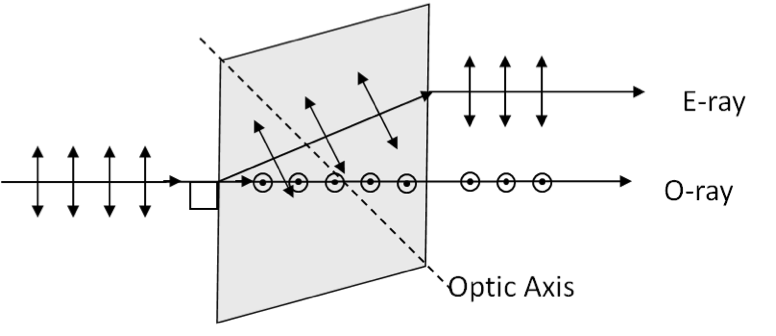## Section49.6Birefrengence

In an isotropic material such as water and glass, the refractive index is same in all directions in the material. But when you have a crystalline material, it may have different refractive indices in different directions. Uniaxial crystals such as calcite (Calcium carbonate), tourmaline (Boron silicate) and quartz (Silicon oxide) have a special direction called the optic axis. These crystals are examples of birefringent crystals.

In birefringent crystals, refractive index of light is different depending on the polarization of the wave relative to the optic axis. When an electromagnetic wave is incident along the optic axis, only one wave comes out of the crystal, but when light is incident at an angle to the optic axis, two waves come out as illustrated in Figure 49.6.1.Figure 49.6.1. Light through a birefringent crystal. A polarized light perpendicular to the optic axis is shown incident on at $90^\circ$ on a principal section of a calcite single crystal. A circle with a dot represent perpendicular-to-the-figure direction.

Clearly, one of these directions is consistent with Snell's law of refraction, and the other is not. The wave in the crystal that obeys Snell's law is called the ordinary or O-ray, and the strangely behaving ray is called the extraordinary or E-ray. Due to the generation of two rays, the O-ray and the E-ray, you see two images when you look through a birefringent crystal as illustrated in Figure 49.6.2.

The refractive index of the ordinary ray $n_o$ may be larger or smaller than the refractive index of the extraordinary ray $n_e\text{.}$ The difference $n_e - n_o$ is called the birefringence of the birefringent crystal. The values of some commonly used birefringent crystals are given in Table 49.6.3.